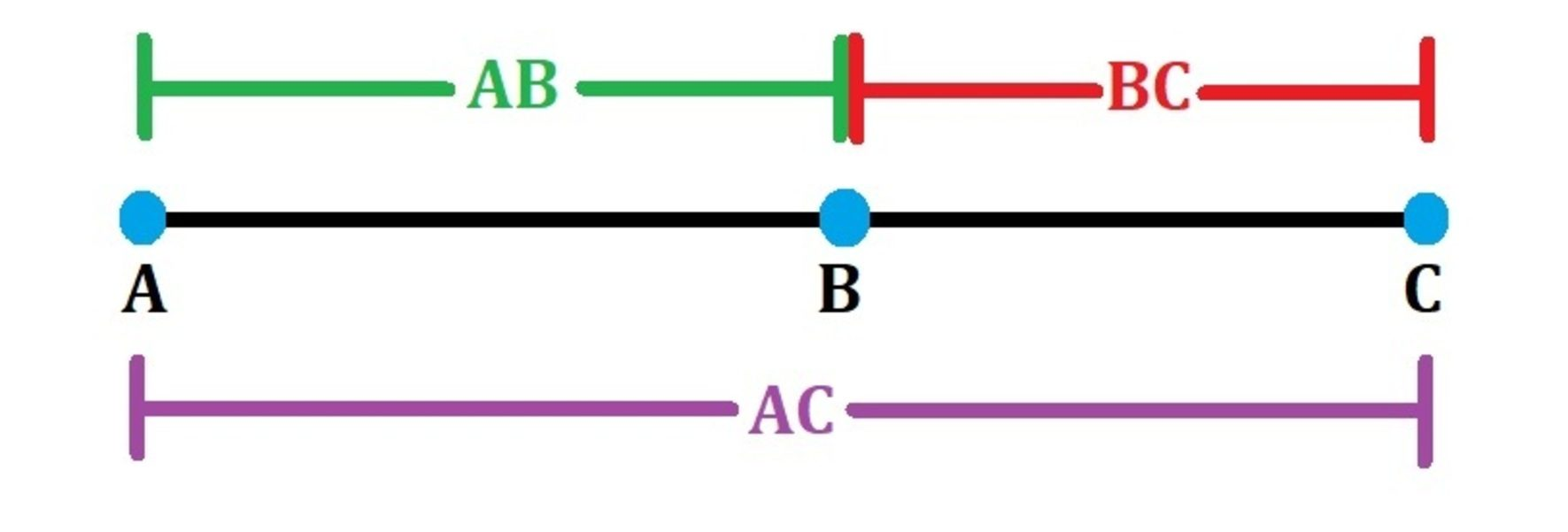Reviewed by Wojciech Sas, PhD candidate and Steven Wooding
Last updated: Nov 12, 2021

The segment addition postulate calculator allows you to apply this property by adding the lengths of two adjacent segments and finding the value of the total segment. We will also see the definition of the segment addition postulate, how the segment addition calculator works, and examples of the segment addition postulate.

## What is the segment addition postulate?

The definition of the segment addition postulate states that if we have a line segment AC and a point B within it, the sum of the lengths of the segments AB and BC will give the total length of AC.

## How do we use the segment addition postulate?

Given a line segment AC and a point B in between, if we know the lengths AB and BC, we can find AC using the equation AC = AB + BC.

The segment addition property (which is based on the distances between points) is used in this segment addition postulate calculator, which also helps you find if a particular point lies on a line segment if the lengths are known.

If the sum of the segments equals the total length, the points must be on the same line, and we say they are collinear. The calculator can check if this is the case by selecting the mode I want to... check if points are collinear.

For instance, in a line segment AC, if you know that AB = 10 cm and BC = 20 cm, here's how you can find the total length of AC:

1. Enter 10 cm for AB in the segment addition postulate calculator;
2. Enter 20 cm for BC; and
3. Voilà! Using the segment addition property, we can see that the total length of AC is 30 cm!

The extra-cool feature is that if you know the total length and the length of one segment, the calculator will automatically find the length of the other segment for you!

## Segment bisector

As a special case, if we know that a segment AC is bisected by point B, then we can see that AB and BC are equal!

So, for instance, if we know that AB is 10 cm, then using the segment bisector property, it would mean BC is also equal to 10 cm, which would give us the value of AC as 20 cm using this segment addition calculator.

There's also a special case of segment division, called the golden ratio, in which the ratio of the total segment to the larger segment, is equal to the ratio of the larger segment to the smaller segment. If the segment lengths follow this property, we say they are in golden ratio, which is approximately equal to 1.618.

## How do we use the segment addition postulate practically?

The practical applications of this segment addition postulate calculator can also be extended to random scenarios as follows:

• You happen to cut a thread into two or more pieces and want to find the total length of the original thread — just add up the individual lengths!

• You have bent a stretch of wire to form a rectangle and want to find the total length of the wire — just add up the lengths of the individual sides!

• You want to split an ultra-long sandwich among your friends for lunch — simply divide the total length of the sandwich by the number of people, and enjoy your share!

Some other interesting applications of addition in different contexts are:

## FAQ

### Can the segment addition postulate be extended to more than two segments?

Yes! If there are multiple points within a line segment, the total sum of each elementary segment will give the total length of the line segment!

### If AB equals 5 and BC equals 10, what is AC?

If the points A, B, C are on the same line, and B lies in between A and C, then AC is the sum of AB and BC. So we'd get AC = 15. On the other hand, if A lies in between B and C, then AC is the difference BC - AB, which gives us AC = 5.

If the points A, B, C are not collinear, then the AC length will depend on the angle that's between AB and BC.I want to…
calculate segment's length.
Enter any two lengths to find the third length
AB
in
BC
in
AC
in
People also viewed…

### Chilled drink

With the chilled drink calculator you can quickly check how long you need to keep your drink in the fridge or another cold place to have it at its optimal temperature. You can follow how the temperature changes with time with our interactive graph.

### Polynomial graphing

The polynomial graphing calculator is here to help you with one-variable polynomials up to degree four. It not only draws the graph, but also finds the functions roots and critical points (if they exist).

### Sum of Series

The sum of series calculator helps you find the sum of an arithmetic or geometric series. Using this tool, you can also find the sum of an infinite geometric series.

### Titration

Use our titration calculator to determine the molarity of your solution.